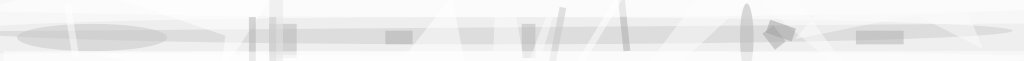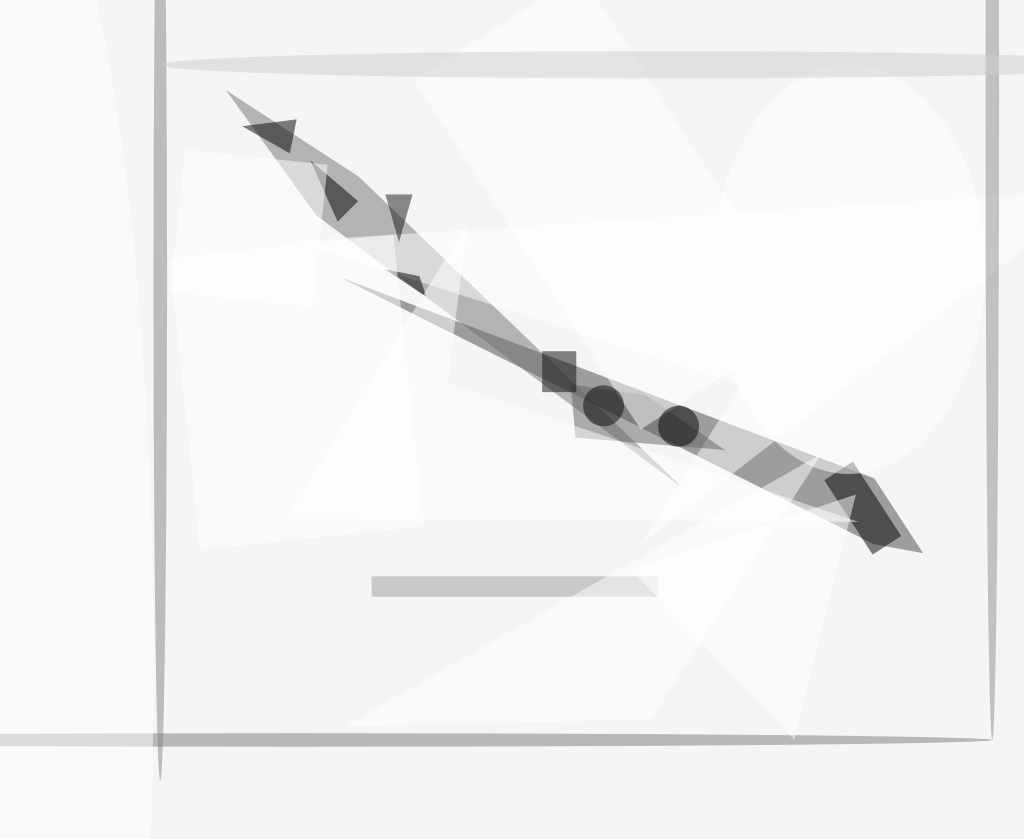MOBILITY ENGINEERING

# Peridynamic Modeling: An Alternative Approach to Analyzing Material Failure

A critical technology challenge for structural material applications in the aerospace and defense industries is to have a means for the reliable analysis of material damage and failure. Experimental structural assessments are typically expensive and often do not provide full information about coupled, multiscale damage processes. Computer-aided analysis has established itself as a useful tool for complementing experimental structural assessments. A comparative summary of current computer-aided approaches is presented in the accompanying table.

The Finite Element Method (FEM) has received widespread acceptance in the computer-aided assessments of the traditional macroscale materials and components. Extensions of classical FEM to model fracture and crack propagation were also developed. Recently, a peridynamic virtual testing that unifies the mechanics of continuous media, cracks, and discrete particles emerged as an enhancement to FEM modeling.

Peridynamic was introduced by Steward Silling in 2000 at Sandia National Laboratories and was further developed by Madenci and Oterkus in 2013, and by Littlewood, Silling, Mitchell, Seleson, Bond, Parks, Turner, Burnett, Ostien, and Gunzburger in 2015. There are several important advantages of the peridynamic method over the FEM. First, it uses displacements rather than displacement derivatives in its formulation. Hence, the peridynamic equations are valid everywhere, including discontinuities. Second, the peridynamic method exploits a length scale variable, to be called horizon, that enables the use of the peridynamic equations at disparate scales. Third, the peridynamic method does not require an external criterion to model crack initiation and propagation since material failure is invoked through the direct material response. Fourth, it discards requirements on mesh size in damage models.

Currently, the peridynamic method has received acceptance in the research community with a steadily increasing number of peer-reviewed papers each year. However, a wide adoption of peridynamic in industry is limited by some unfavorable factors such as a lack of integration with mainstream FEM software, a relatively high computational cost, and a scarcity of demonstration for important engineering applications.

#### The Peridynamic Method

The peridynamic methodology pursued in this study is a nonlocal theory based on interactions between material points. Here, points are connected by imaginary mechanical bonds and can interact with each other according to nonlocal forces. The peridynamic equation of deformation is given by (Littlewood et at, 2016):where T is the nonlocal force, x is a material point in the reference configuration of a body B, u is the deformation, ρ is the density field, and b is the prescribed external body force density. The integration in (1) is over a spherical neighborhood in B centered at x. It can be demonstrated that Equation (1) will recover the classical Cauchy momentum equation if the integral of nonlocal forces is replaced with the divergence of stress.

Existing peridynamic material models can be classified into the following three categories. The first category is bond-based material models that are computationally inexpensive and robust but account only for simple two-point interactions with Poisson’s ratio of one quarter. The second category is ordinary state-based material models in which the pairwise force between any two material points depends on the deformations of all material points within the neighborhood of these two points. The third category represents non-ordinary state-based material models providing a way to use material models developed for the FEM within a peridynamic framework.

Different types of bonds can be included in a peridynamic damage model, which stretch arbitrarily in any direction and fail in different ways. Currently, the peridynamics code MesoEqs, utilized in this study, supports two bond damage models: a critical stretch model and a fatigue damage model. The critical stretch model uses a critical stretch bond breaking criterion with critical stretch parameters determining when each individual bond is broken. The critical stretch parameters can be calibrated using experimentally measured energy release rates. The fatigue damage model follows an approach by Silling and Askari, 2014, that does not require any pre-defined criterion in its formulation. Here, the associated remaining life D at any given time t for each bond is determined by expression (2) below:where τ = Δt/ln(f Δt), s is the cyclic bond strain, Δt is the time step of peridynamic solver, R is the loading ratio, A is the fitting coefficient, m is the fitting degree, and f is the frequency of rotation per minute. The cyclic strain is related to the cyclic stress intensity factor ΔK through expression (3):where E is the modulus, δ is the horizon, and g(r/ δ) is a function. Fatigue life is known to highly depend on the quality of surface. This effect can be accounted for in the damage model by rescaling the cyclic stress intensity factor as following [see expression (4)]:where the “r” and “s” in the superscript stands for rough and smooth surfaces, respectively, Kt is a factor that can depend on the average roughness (Ra), the peak-to-valley height roughness (Ry), and the 10-point roughness (Rz), the effective profile valley radius of the surface texture (q), and the stress state for tension. Considering Equations (3) and (4), the cyclic strains for rough and smooth surfaces can be related as [see expression (5)]:Equation (5) can be directly applied to compute remaining life D for each bond near the rough surface during peridynamic modeling. Such bonds need to be ether tracked during the simulation or picked based on the number of nearest neighbors as currently implemented in MesoEqs. Indeed, nearest neighbors are material points that are located within a sphere of radius equal to horizon. Surface points are expected to have a smaller number of neighbors compared to those in bulk. Consequently, Equation (5) will be effective only to those points with a number of neighbors less than a chosen criterion.

#### FEM/Peridynamic Modeling

One of the promising approaches to circumvent the shortcomings of the peridynamic method is to use this method only in regions susceptible to material failure while using the FEM elsewhere. A two-tier bridging framework for coupling the FEM simulation in local domains with the peridynamic modeling in nonlocal regions was developed to demonstrate the feasibility of such a concept. Here, a peridynamic partial-stress technique was used in the transitions regions to greatly reduce the mathematical incompatibilities between the local and nonlocal equations through reduction of the peridynamic horizon near interfaces between the local and nonlocal domains.

Feasibility of the bridging framework was demonstrated by blending mainstream ACE+ Multiphysics software by ESI-group with the peridynamic solver, to be called MesoEqs. A bar under tension test problem was considered. The bar was discretized into five domains: a mesh-free peridynamic domain in the center, two FEM regions at the ends of the bar, and two peridynamic partial-stress regions in-between. FEM and peridynamic simulations were performed with the elastic and elastic correspondence material models, respectively. Modeling results are presented in Figure 1.

Smooth transitions between solutions obtained using FEM, the peridynamic partial-stress approach, and the mesh-free peridynamic validated the two-tier bridging framework. As discussed above, one of the limiting factors for the adoption of peridynamic in industry is a relatively high computational cost that is 3× to 5× higher than the standard FEM computational cost. The feasibility of improving the efficiency of peridynamic modeling by separating the modeling domain into local and nonlocal regions was examined for a large-scale tensile test problem. It was determined that the coupled FEM/peridynamic modeling was 1.6× faster than the classical peridynamic simulation when the horizon was 6-times larger than the peridynamic node spacing, a typical situation for modeling systems with material damage.

#### High-Cycle Fatigue Modeling

Turbine blades of aircraft engines can be responsible for up to 75% of an aircraft's thrust. The high-cycle fatigue (HCF) failure of the turbine blades has traditionally been analyzed with a Goodman diagram. However, the high incidence of HCF related failures calls for new approaches such as peridynamic.Figure 2. Analytical fit to a strain ratio versus cycle dependence was developed to calibrate the peridynamic fatigue damage model. Experimental data by Zhao and Jiang, 2008 for a 7075-T651 aluminum alloy were used.

The peridynamic MesoEqs software was applied to the modeling of a turbine blade made from 7075-T651 aluminum alloy. Coefficients required for computing the remaining life in the peridynamic HCF damage model given by Equation 2 were obtained by developing an analytical fit to a strain ratio versus cycle dependence reported by Zhao and Jiang in 2008 for that alloy. The obtained fit is shown in Figure 2.